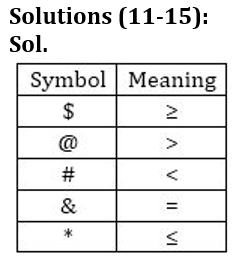Latest Banking jobs   »

# Reasoning Ability Quiz For IDBI AM/ Bank of India PO 2023 -14th March

Directions (1-5): Study the following information carefully to answer these questions.

M R 1 5 % ? 6 8 3 D K \$ T H 9 * F 2 P 7 # @ 4 J

Q1. How many such numbers are there in the above arrangement which are immediately followed by other number and immediately preceded by a consonant?
(a) None
(b) Three
(c) Two
(d) One
(e) None of these

Q2. How many such symbols are there in the above arrangement which are immediately followed by a symbol and also immediately preceded by a number?
(a) Two
(b) Three
(c) One
(d) Four
(e) None of these

Q3. What will come in place of ‘__’ in the following series based on the above arrangement?
M51 ?38 KHT __
(a) 72P
(b) *P2
(c) 2P*
(d) *2P
(e) None of these

Q4. Which of the following element is 15th to the left of the one which is 21st from the left end of the given arrangement?
(a) R
(b) %
(c) 5
(d) ?
(e) None of these

Q5. How many such consonants are there in the above arrangement which are immediately followed by a number but not immediately preceded by a symbol?
(a) One
(b) Two
(c) Three
(d) None
(e) None of these

Directions (6-10): Study the following sequence and answer the given questions.

K & M 3 9 U # T Q 2 ! H 7 D * 8 Y E 5 A \$ @ G S 4 5 R ^ 0 % W 1 O

Q6. Which of the following element is 10th to the left of the one which is 21st from the left end of the given arrangement?
(a) !
(b) H
(c) 2
(d) ^
(e) None of these

Q7. If all the digits are dropped from the series, which element will be tenth from the right end of the new arrangement?
(a) &
(b) @
(c) \$
(d) A
(e) None of these

Q8. How many such symbols are there in the given series which are immediately preceded by a number and immediately followed by a consonant?
(a) None
(b) One
(c) Two
(d) Three
(e) Four

Q9. How many such number are there in the given series which are immediately preceded by number and immediately followed by symbol?
(a) One
(b) Two
(c) Three
(d) More than three
(e) None of these

Q10. What should come in place of question mark (?) in the following series based on the above arrangement?
KO MW 90 ?
(a) R#
(b) K3
(c) &3
(d) #R
(e) None of these

Directions (11-15): In the following questions, the symbols @, \$, *, # and & used with the following meaning as illustrated below:
‘P \$ Q’ means ‘P is not smaller than Q’
‘P@Q’ means ‘P is neither smaller than nor equal to Q’
‘P # Q’ means ‘P is neither greater than nor equal to Q’
‘P&Q’ means “P is neither greater than nor smaller than Q’
‘P *Q’ means ‘P is not greater than Q’
Now, in each of the following questions assuming the given statements to be true, find which of the three conclusions I, II and III is/are definitely true and give your answer accordingly.

Q11. Statements: L & M \$ T # U* P
Conclusions: I. M @ T
II. U @ P
III. T & P
(a) None is true
(b) Only I is true
(c) Only II is true
(d) Only III is true
(e) Only I and III true

Q12. Statements: F # U @ G, C * D, F * D
Conclusions: I. F @ G
II. D @ U
III. C # U
(a) None is true
(b) Only I is true
(c) Only II is true
(d) Only III is true
(e) Only I and II true

Q13. Statements: P @ S # N & H, Y \$ H
Conclusions: I. N * Y
II. H @ S
III. P @ Y
(a) None is true
(b) Only I is true
(c) Only II is true
(d) Only III is true
(e) None of these

Q14. Statements: X * U, W # V, S \$ V, S @ U
Conclusions: I. V # U
II. X # S
III. X # V
(a) Only II and III are true
(b) Only III and I are true
(c) Only I and II are true
(d) All are true
(e) Only II is true.

Q15. Statements: H * L, H # F, M @ L, C \$ F
Conclusions: I. F @ M
II. C \$ L
III. M @ F
(a) Only I and II are true
(b) Only I and III are true
(c) Only I are true
(d) Only III is true
(e) None of these

Solutions

Solutions (1-5):
S1. Ans.(d)
Sol. R15
S2. Ans.(a)
Sol. 7#@, 5%?
S3. Ans.(b)
S4. Ans.(d)
S5. Ans.(c)
Sol. 2P7, TH9, MR1

Solutions (6-10):
S6. Ans. (a)
S7. Ans. (d)
S8. Ans. (c)
Sol. Two i.e. 0%W 2!H
S9. Ans. (e)
S10. Ans. (d)S11. Ans.(a)
Sol.
(I) M > T(false)
(II) U > P(false)
(III) T = P(false)

S12. Ans.(a)
Sol.
(I) F > G (false)
(II) D > U (false)
(III) C < U (false)

S13. Ans (e)
Sol.
(I). N ≤Y (true)
(II). H > S(true)
(III). P > Y (false)

S14. Ans.(e)
Sol.
(I) V < U (false)
(II) X < S (true)
(III) X < V (false)

S15. Ans.(e)
Sol.
(I) F > M(false)
(II) C ≥ L (false)
(III) M > F(false)## FAQs

### What is the selection process of the IDBI AM?

The selection process of IDBI AM is Online exam and Interview.

#### Congratulations!Union Budget 2023-24: Free PDF